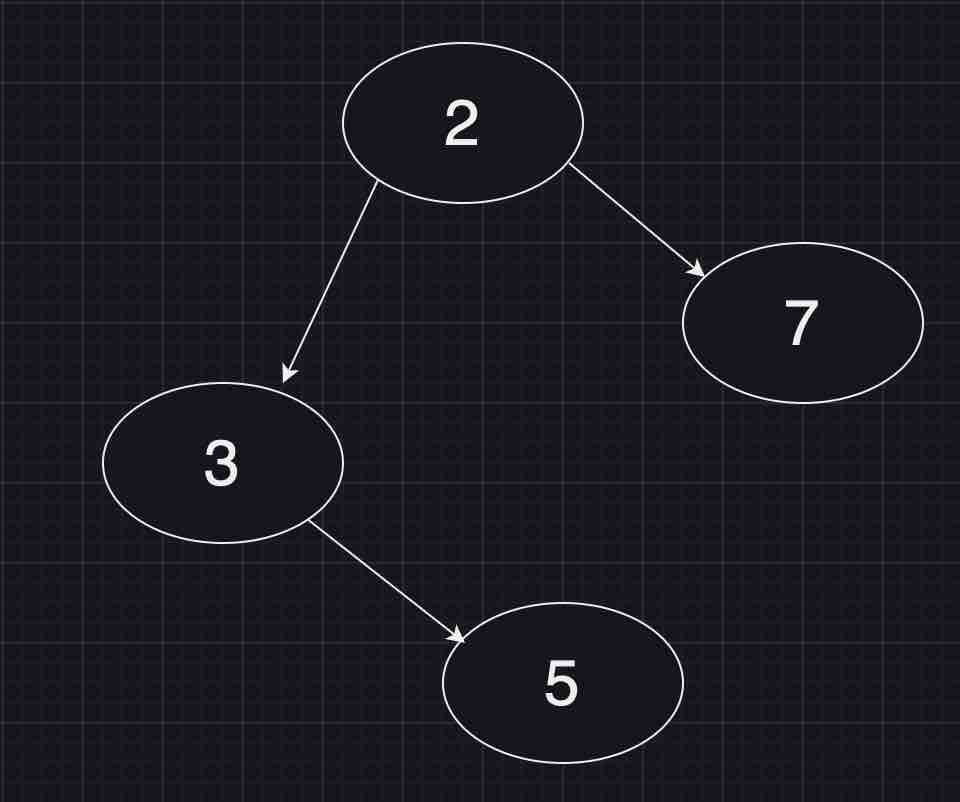Paul Coroneos
Published on

# Leetcode 701. Insert into a binary search tree

Today we will be going over Leetcode 701 - Insert into a binary search tree. This is a classic DFS (depth-first search) problem we will implement using recursion.

## Problem Statement and Analysis

You are given the root node of a binary search tree (BST) and a value to insert into the tree. Return the root node of the BST after the insertion. It is guaranteed that the new value does not exist in the original BST.

Notice that there may exist multiple valid ways for the insertion, as long as the tree remains a BST after insertion. You can return any of them.

As previously stated this is a classic DFS problem. What makes it unique is that there are multiple possible answers per tree (this will not always be the case, such as in the case of requiring the tree to be balanced. We will likely get to this problem in the future when I am more comfortable with BST.) In other words, this problem is fine as long as we satisfy the property of BST's in that all nodes to the left of a node are less than or equal to the node and all nodes to the right of a node are greater than the node.

Let's take a look at a sample BST:Let's say for example we want to insert the value 6 into the above BST. We can either insert it to the left of node 7 or the right of node 5. Both of these are valid answers. So how would we approach doing this recursively? First, we should define our base case. If the root node is null we would want to return the node we are intending to insert (6). But where else does this base case come into play? What is common in the above two previous examples? If you said nodes 5 and 7 are both leaf nodes you are correct! So in other words the second usage of the base case is when we try to navigate to the left or right of a leaf node. In this case, we should just insert the new node and we are done!

We will now just follow the standard recursive navigation of a BST. If the value at the current node is larger than the new node val, we go left. Otherwise, we need to go right (the problem guarantees the inserted node is unique so we don't have to worry about the equal case). There is one small additional trick to consider here. Since we want to perform insertion in this case we should not just navigate to either the right or left child. Rather we will assign the right or left child to point to our recursive call. Why? Well, again we are potentially creating a new tree when we go left or right (the new tree is created when we return the new node). If we take a step back a BST is a composition of many smaller BST. So at the end of the day, we are creating references to smaller BST trees through recursion by assigning the left or right child to the result of our recursive call. Since we are returning root to satisfy the problem statement we need this continuity otherwise we would have no reference to the newly updated tree.

With that all out of the way let's take a look at the implementation using JavaScript:

``````/**
* Definition for a binary tree node.
* function TreeNode(val, left, right) {
*     this.val = (val===undefined ? 0 : val)
*     this.left = (left===undefined ? null : left)
*     this.right = (right===undefined ? null : right)
* }
*/
/**
* @param {TreeNode} root
* @param {number} val
* @return {TreeNode}
*/

var insertIntoBST = function(root, val) {
const newNode = new TreeNode(val)
if(!root) return newNode;
if(val > root.val) root.right = insertIntoBST(root.right,val)
else root.left = insertIntoBST(root.left,val)
return root;
};
``````

## Time and Space Complexity Analysis

As with any tree problem, we have a worst-case time complexity of O(N) (where N is the number of tree nodes) since there is no guarantee the tree is balanced. Or in other words, every node in the resulting tree can be on a different level. The space complexity is similarly O(N) because we could place recursive calls to every node of the tree on the call stack in the aforementioned scenario. The average case for both would be O(logN) if the tree has any semblance of balance (meaning the tree has nodes distributed somewhat evenly across levels).

Thank you for reading my blog post and best of luck in your learning!